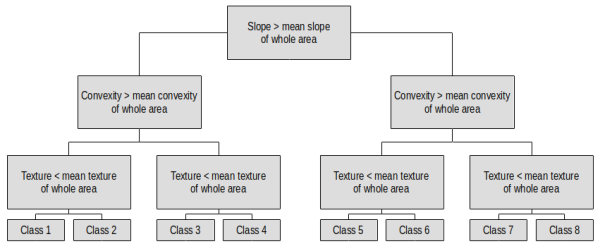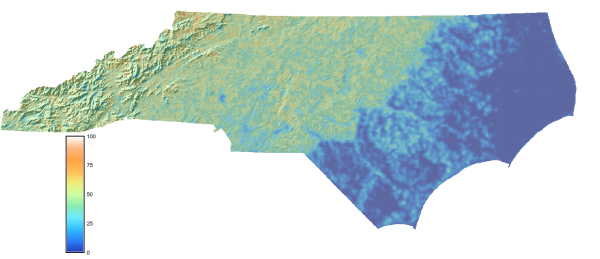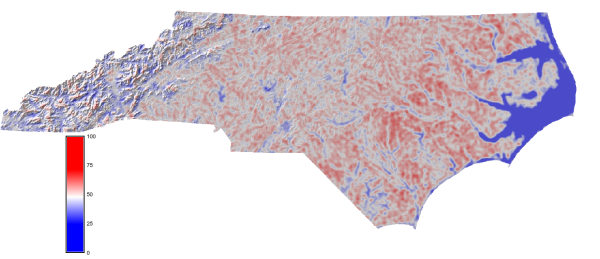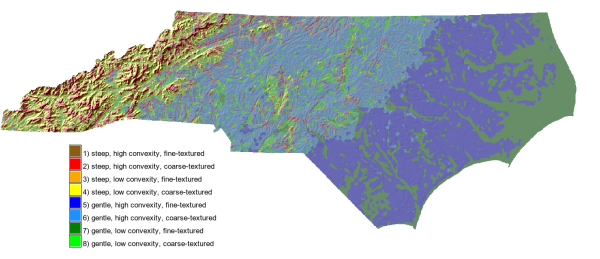## NAME

r.terrain.texture - Unsupervised nested-means algorithm for terrain classification

## KEYWORDS

raster, terrain, classification

## SYNOPSIS

r.terrain.texture
r.terrain.texture --help
r.terrain.texture elevation=name [slope=name] [flat_thres=float] [curv_thres=float] [filter_size=integer] [counting_size=integer] [classes=integer] texture=name convexity=name concavity=name [features=name] [--overwrite] [--help] [--verbose] [--quiet] [--ui]

### Flags:

--overwrite
Allow output files to overwrite existing files
--help
Print usage summary
--verbose
Verbose module output
--quiet
Quiet module output
--ui
Force launching GUI dialog

### Parameters:

elevation=name [required]
Input elevation raster:
slope=name
Input slope raster:
flat_thres=float
Height threshold for pit and peak detection:
Default: 1
curv_thres=float
Curvature threshold for convexity and concavity detection:
Default: 0
filter_size=integer
Size of smoothing filter window:
Default: 3
counting_size=integer
Size of counting window:
Default: 21
classes=integer
Number of classes in nested terrain classification:
Options: 8, 12, 16
Default: 8
texture=name [required]
Output terrain texture:
convexity=name [required]
Output terrain convexity:
concavity=name [required]
Output terrain concavity:
features=name
Output terrain classification:

## DESCRIPTION

r.terrain.texture calculates the nested-means terrain classification of Iwahashi and Pike (2007). This classification procedure relies on three surface-form metrics consisting of: (a) terrain surface texture which is represented by the spatial frequency of peaks and pits; (b) surface-form convexity and concavity which are represented by the spatial frequency of convex/concave locations; and (c) slope-gradient which should be supplied by r.slope.aspect or r.param.scale. These metrics are combined using the mean of each variable as a dividing measure into a 8, 12 or 16 category classification of the topography.

The calculation follows the description in Iwahashi and Pike (2007). Terrain surface texture is calculated by smoothing the input elevation using a median filter over a neighborhood specified by the filter_size parameter (in pixels). Second, pits and peaks are extracted based on the difference between the smoothed DEM and the original terrain surface. By default the algorithm uses a threshold of 1 (> 1 m elevation difference) to identify pits and peaks. This threshold is set in the flat_thres parameter. The spatial frequency of pits and peaks is then calculated using a Gaussian resampling filter over a neighborhood size specified in the counting_filter parameter (default is 21 x 21 pixels, as per Iwahashi and Pike (2007).

Surface-form convexity and concavity are calculated by first using a Laplacian filter approximating the second derivative of elevation to measure surface curvature. The Laplacian filter neighborhood size is set by the filter_size parameter (in pixels). This yields positive values in convex-upward areas, and negative values in concave areas, and zero on planar areas. Pixels are identified as either convex or concave when their values exceed the curv_thres. Similarly to terrain surface texture, the spatial frequency of these locations is then calculated to yield terrain surface convexity and concavity measures.

Optionally, these surface-form metrics along with slope-gradient can be used to produce a nested-means classification of the topography. Each class is estimated based on whether the pixels values for each variable exceed the mean of that variable. The classification sequence follows:A single iteration of this decision tree is completed for the 8-category classification. However for the 12 category classification, classes 1-4 remain but pixels that otherwise relate to classes 5-8 are passed to a second iteration of the decision tree, except that the mean of the gentler half of the area is used as the decision threshold, to produce 8 additional classes. Similarly for the 16 category classification, pixels that otherwise relate to classes 8-12 are passed onto a third iteration of the decision tree, except that the mean of the gentler quarter of the area is used as the decision threshold. This iterative subdivision of terrain properties acts to progressively partition the terrain into more gentle terrain features.

## NOTES

In the original algorithm description, SRTM data was smoothed using a fixed 3 x 3 size median filter and the spatial frequency of extracted features were measured over a 21 x 21 sized counting window. However, a larger smoothing filter size (~15 x 15) is often required to extract meaningful terrain features from higher resolution topographic data such as derived from LiDAR, and therefore both filter_size and counting_size parameters were exposed in the GRASS implementation. Further, if a large filter size is used then the counting window size should be increased accordingly.

## EXAMPLE

Here we are going to use the GRASS GIS sample North Carolina data set as a basis to perform a terrain classification. First we set the computational region to the elev_state_500m dem, and then generate shaded relief (for visualization) and slope-gradient maps:

```g.region raster=elev_state_500m@PERMANENT
r.slope.aspect elevation=elev_state_500m@PERMANENT slope=slope_state_500m
```

Then we produce the terrain classification:

```r.terrain.texture elevation=elev_state_500m@PERMANENT slope=slope_state_500m@PERMANENT \
texture=texture_state_500m convexity=convexity_state_500m concavity=concavity_state_500m \
features=classification_state_500m
```

Terrain surface texture:Surface-form convexity:8-category terrain classification:## REFERENCES

Iwahashi, J., and Pike, R.J. 2007. Automated classifications of topography from DEMs by an unsupervised nested-means algorithm and a three-part geometric signature. Geomorphology 86, 409-440.

Steven Pawley

## SOURCE CODE

Available at: r.terrain.texture source code (history)

© 2003-2020 GRASS Development Team, GRASS GIS 7.8.4dev Reference Manual